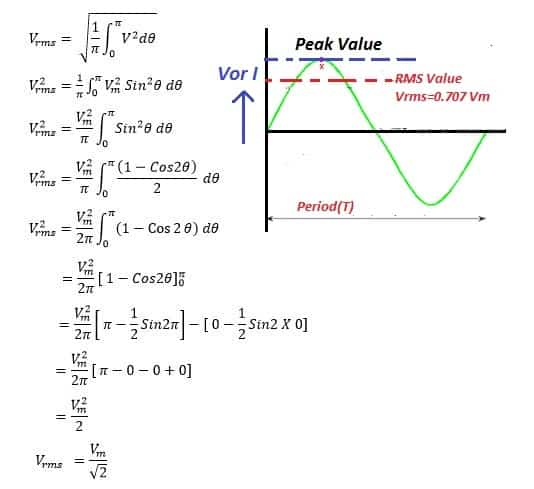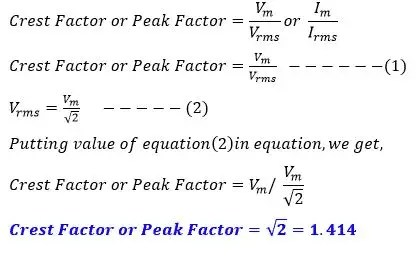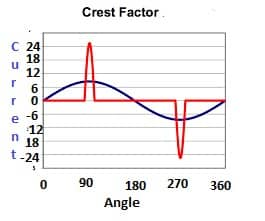# What is Crest Factor or Peak Factor? Its formula & Derivation

The ratio of Peak value to root mean square(RMS) value of AC quantity is called Crest Factor or Peak Factor. Any periodic waveform has its varying magnitude with time. The waveform attains maximum or peak value at a point of time. The direct current has its maximum amplitude at all the times i,e. it has constant magnitude  and the magnitude does not change with time. In case of the alternating current, the instantaneous value varies with time and attains maximum or peak value and again the magnitude gets decreased.

What is Crest Factor?

The crest factor of an AC current or voltage waveform is the ratio of its peak value to root mean square(RMS) value.The crest factor of an AC voltage or current can be found by measuring its peak value and root mean square value.The root mean square value of an AC current is equal to the heating effect produced by the AC current that is equal to the heating effect produced by the DC current. The root mean square value of an perfect sinusoidal AC voltage or current has certain relationship with its peak or maximum value.

### RMS Value of AC Waveform

The RMS value of an AC voltage is as given below.## Crest Factor Formula Derivation

The crest factor of an AC current or voltage waveform can be mathematically expressed as;### Importance of Crest Factor

The crest factor 1 indicates no peaks.Higher crest factor indicates peaks.The crest factor of DC current or voltage is equal to unity because RMS value of the DC is equal to the peak value.
The crest factor of the perfect sinusoidal voltage or current is 1.414. The crest factor of AC other than 1.414 shows that the waveform is not sinusoidal.

When the sinusoidal voltage is fed to semiconductor devices the current through the devices is not linear and the current is non sinusoidal. The crest factor of the current drawn by the semiconductor devices is much higher than 1.414.

Crest factor different than 1.414 indicates distortion in waveform. Typically distorted current waveform have crest factors higher than 1.414 or may have lower than 1.414. Distorted voltage waveform with crest factor lower than 1.414 are called  flat top  voltage waveform.

The Computer and Business Equipment Manufacturing Association (CBEMA) recommends a method for de-rating transformers based on the current crest factor. The de-rated KVA of the transformer would be the nominal KVA of the transformer multiplied by total harmonic distortion factor(THDF).

### Crest Factor of sinusoidal and non sinusoidal waveformFrom above figure;
Crest Factor of sinusoidal waveform

Crest Factor= I peak/ Irms= 8.20/5.8=1.414

Crest Factor of non sinusoidal waveform

Crest Factor= I peak/ Irms= 24.2/5.8=4.17

### Significance of Crest Factor

#### Power Supply Capacity  Selection

The rating of power supply is decided on the basis of the peak current demanded by the load. The higher crest factor loads demands much higher capacity power supply or the supply need to be de-rated.

#### Current Transformer(CT) Selection

If the peak current is too high, the metering CT inputs can clip, causing inaccurate readings.This means that when measuring loads with high current crest factors, CT current rating needs to be selected considering crest factor of the load. For example, if your load draws 15 amps RMS, but has a crest factor of 4.0, then the peak current is 60 amps. If you use a 20 amp CT, the meter will not be able to accurately measure the 30 amp peak current.

The following graph shows the maximum RMS current for accurate measurements as a function of the current waveform crest factor. The current is shown as a percentage of CT rated current. For example, if you have a 10 amp load with a crest factor of 3.0, the maximum CT current is approximately 58%. Fifty-eight percent of 20 amps is 11.60, which is higher than 10 amps, so your measurements should be accurate.

On the other hand, if you have a 100 amp load with a crest factor of 4.0, the maximum CT current is 42%. Forty-two percent of a 250 amp CT is 105 amps, so you would need a 250 amp CT to accurately measure this 100 amp load.

If crest factor of the load is not known, the crest factor can be assumed to be in the range in 1.4 to 1.5 and CT current rating 150% of the expected RMS current can be selected for  measurement.So if the measuring currents up to 50 amps, select a 75 amp CT.